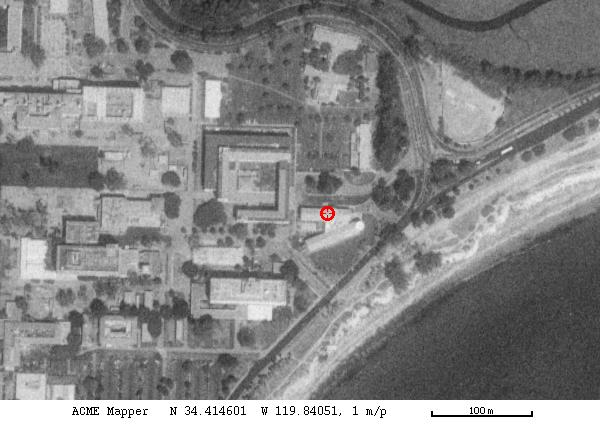## July 25, 2003

### Location, Location, Location

Here’s my office at the KITP (on a less foggy day and minus the construction, which is currently causing the building to shake).Ron Donagi gave a great talk this morning about a version of Dijkgraaf-Vafa and large-N duality for compact Calabi-Yau’s. DV’s setup can be thought of as a noncompact Calabi-Yau

(1)$y^2 = u v +W'(x)^2$

which, generically, has $n$ isolated $A_1$ singularities at $u=v=y=W'(x)=0$. If $W(x) = \sum_i^0^{n+1} a_i x^i$, these can be smoothed by adding $f_\mu(x)=\sum_{i=0}^{n-1}\mu_i x^i$

(2)$y^2 = u v +W'(x)^2 +f_\mu(x)$

The salient feature, according to Ron is that this degenerates to a curve (the plane $\{ u=v=y=0\}$) when the parameters $a=\mu=0$.

Their compact example is the intersection of a quadric and a quartic in $\mathbb{C}P^5$. When the quadric has rank 3, one has a genus-3 curve of $A_1$ singularities, while the generic quadric gives a smooth Calabi-Yau. To first order in the deformation, they compute the corresponding integrable system and solve for the open-string invariants.

It’s quite a tour de force, made all the more remarkable by the fact that it’s not clear what they are calculating. Since the Calabi-Yau is compact, the total D-brane charge must vanish. So they need to put in both $N$ and $N$ antibranes (on the open string side). In a supersymmetric theory, the quantity they calculate would be the related to the glueball superpotential. In this non-supersymmetric gauge theory, I’m not sure what the interpretation should be. But it’s pretty exciting if there’s something exact that you can calculate in this nonsupersymmetric theory.

Greg Moore also gave a great talk about the C-field in M-theory. Yup, it’s an element of differential cohomology, $\check{H}^4(X)$. Mike Hopkins gave a series of lectures last week touching on this, which went way over my head. But these guys actually have a concrete model one can wrap one’s head around.

The idea is that, up to 15-skeletons, the classifying space of $E_8$ bundles is an Eilenberg-MacLane space

(3)$BE_8 \sim K(\mathbb{Z}, 4)$

So an isomorphism class of $E_8$ bundle on an 11-manifold is equivalent to a choice of cohomology class $a\in H^4(X,\mathbb{Z})$. Their concrete model for the C-field is a pair $(A,c)$, consisting of connection on an $E_8$ principal bundle, $P$, and an honest-to-God, globally-defined, 3-form $c$. They can write explicit formulas for the bosonic part of the 11D supergravity action in terms of $(A,c)$ and the field strength,

(4)$G = d c + tr F^2 - \textstyle{\frac{1}{2}} tr R^2$

which are invariant under gauge transformations

(5)$(A,c) \to ( A + \alpha, c- CS(A,A+\alpha)+\omega)$

where $(\alpha,\omega) \in \Omega^1( ad P)\times \Omega^3_{\mathbb{Z}}(X)$. Naively, the gauge group is $\Omega^1( ad P)\ltimes \Omega^3_{\mathbb{Z}}(X)$, with the composition law

(6)$(\alpha_1,\omega_1)\cdot (\alpha_2,\omega_2)=(\alpha_1+\alpha_2, \omega_1+\omega_2+ d (tr \alpha_1\wedge\alpha_2) )$

Now, Greg says this is too naive, and one should really take the gauge group to be $\Omega^1( ad P)\ltimes \check{H}^3(X)$ which is a nontrivial extension

(7)$0\to H^2(X,U(1))\to \check{H}^3(X) \to \Omega^3_{\mathbb{Z}}(X)\to 0$

That means I still have to understand differential $H^3$, understand how to compose two such guys, etc.

Rats!

Posted by distler at July 25, 2003 3:04 AM

TrackBack URL for this Entry:   https://golem.ph.utexas.edu/cgi-bin/MT-3.0/dxy-tb.fcgi/193